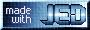# Loving ISIS - Confessions of a Former XSPEC User

Systematic Errors and Custom Statistics

ISIS allows a fair amount of flexibility over the way statistics are calculated and errors are propagated. As in XSPEC, you don't have to think about this if you don't want to, but if you do want to, there's several choices that you can make:

• You can decide what minimization method to use. This is discussed more here.
• You can decide what fit statistic to minimize: chi squared, cash, or a user defined statistic.
• For the chi squared statistic, you can decide which measure of the variance you use: taken from the data, the gehrels error calculated from the binned counts, taken from the model counts, a uniform variance of 1 (lsq), or a user defined error statistic.
• The default statistic is chi squared, with a variance defined to be the binned data counts (i.e., the error being square root of binned counts -- simple Poisson statistics). However, you can redefine how errors are propagated for a rebinned dataset.
Some of the ISIS intrinsic functions designed to allow you to make these choices are:
```     set_fit_method("choice");                 %  Choose minimization method ...
set_fit_statistic("choice");              %  Choose chi squared or cash ...
set_rebin_error_hook(id,"name");          %  Propagate errors your own way
```
Most people most of the time seem to be happy with chi squared statistics with error bars defined by Poisson statistics in each data bin (i.e., the ISIS and XSPEC defaults). In this example, a notable exception is for the high count rate PCA statistics, which are dominated by systematic uncertainties.

There are no truly universally accepted and proper ways of dealing with systematic errors. ISIS will treat them essentially the way that XSPEC does. For a given bin, ISIS will calculate the mean input fractional systematic error for that bin. This error will then be added in quadrature to the statistical error. This will happen automatically if systematic errors are defined in the PHA file.

To remove the systematic errors on dataset `id`, one simply does:

```     set_sys_err_frac(id,NULL);
```
To change the systematic errors on dataset `id`, one simply inputs a wavelength ordered array with the fractional systematic errors. Here is how we add 0.5% systematic errors to the PCA data:
```     set_sys_err_frac(id,Double_Type+0.005);
```
(There are 129 data bins in the PCA spectrum. `Double_Type` initializes to 0 a double precision array of 129 elements.)

ISIS does set a lower bound to the minimum size of a statistical error bar (to avoid roundoff errors, etc.). We violate this lower bound when defining the radio data as a counts/bin dataset. Thus, we overwrite that choice in my `.isisrc` files by setting:
```     Minimum_Stat_Err = 1.e-30;